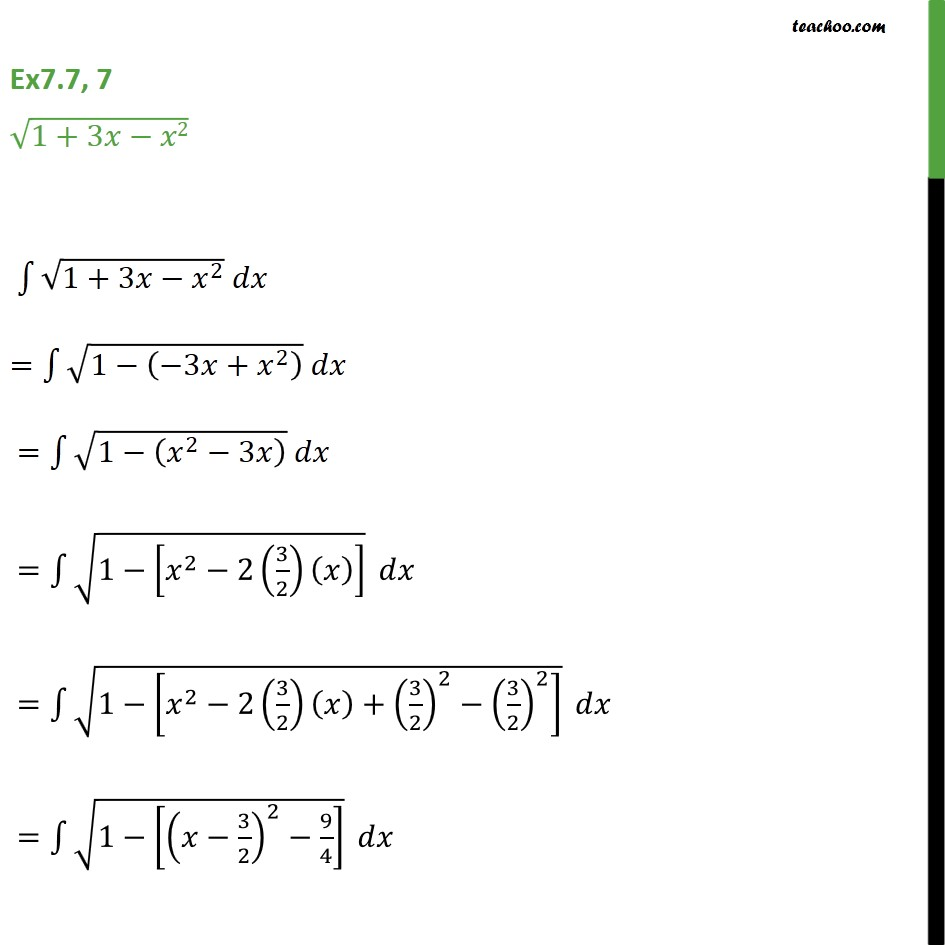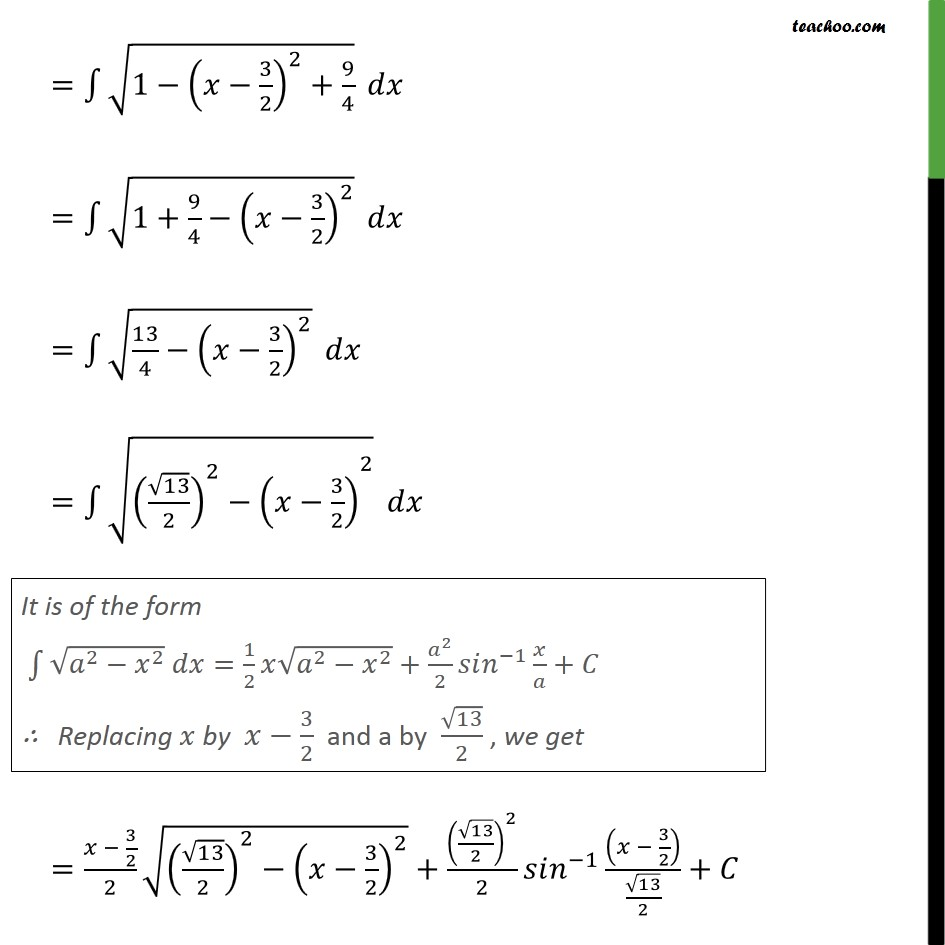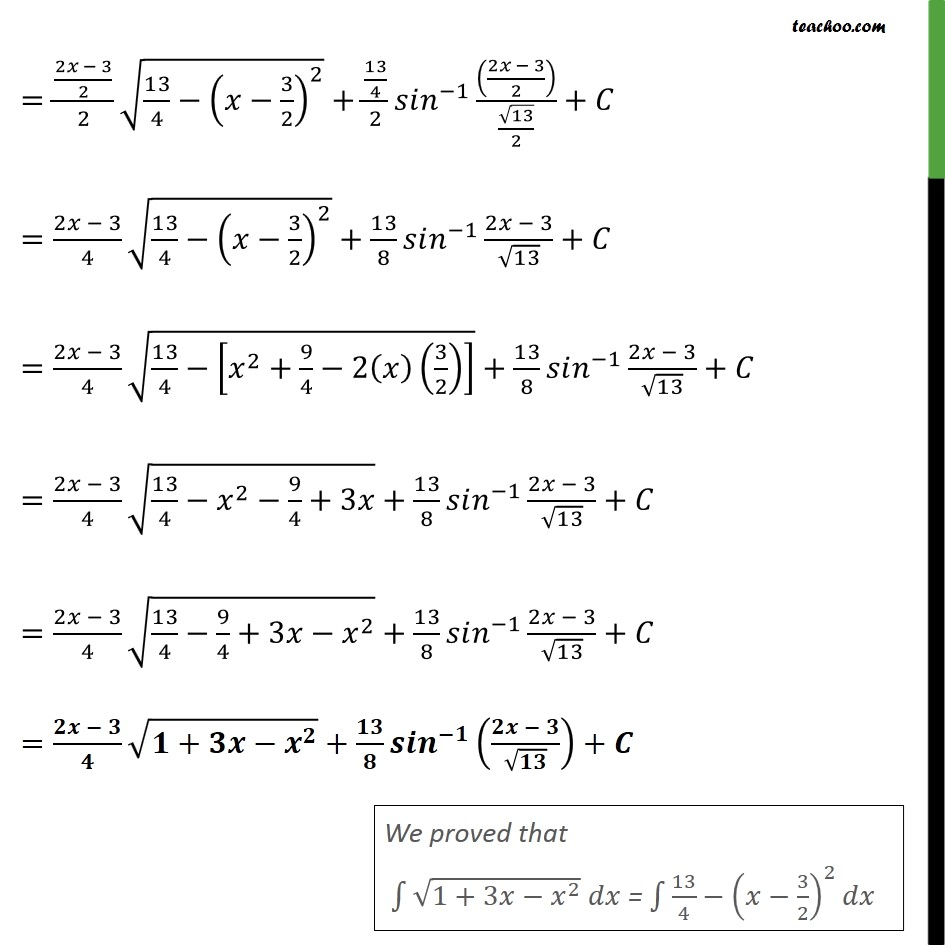1. Chapter 7 Class 12 Integrals
2. Serial order wise
3. Ex 7.7

Transcript

Ex7.7, 7 1+3 2 1+3 2 = 1 3 + 2 = 1 2 3 = 1 2 2 3 2 = 1 2 2 3 2 + 3 2 2 3 2 2 = 1 3 2 2 9 4 = 1 3 2 2 + 9 4 = 1+ 9 4 3 2 2 = 13 4 3 2 2 = 13 2 2 3 2 2 = 3 2 2 13 2 2 3 2 2 + 13 2 2 2 1 3 2 13 2 + = 2 3 2 2 13 4 3 2 2 + 13 4 2 1 2 3 2 13 2 + = 2 3 4 13 4 3 2 2 + 13 8 1 2 3 13 + = 2 3 4 13 4 2 + 9 4 2 3 2 + 13 8 1 2 3 13 + = 2 3 4 13 4 2 9 4 +3 + 13 8 1 2 3 13 + = 2 3 4 13 4 9 4 +3 2 + 13 8 1 2 3 13 + = + + +

Ex 7.7

Chapter 7 Class 12 Integrals
Serial order wise